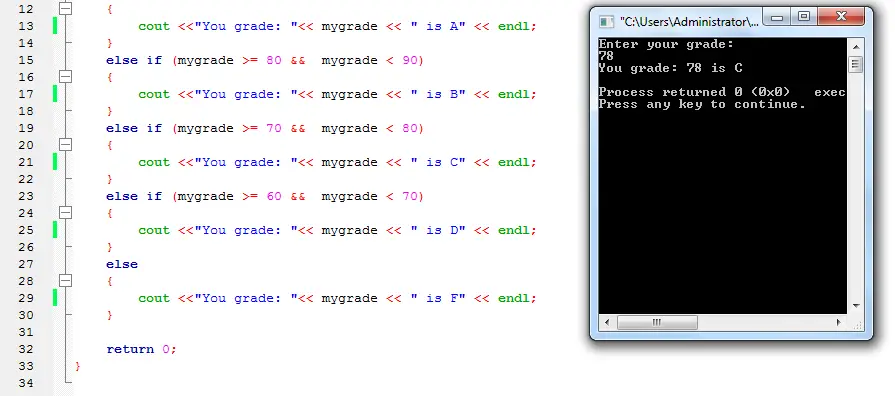## While Loop in C++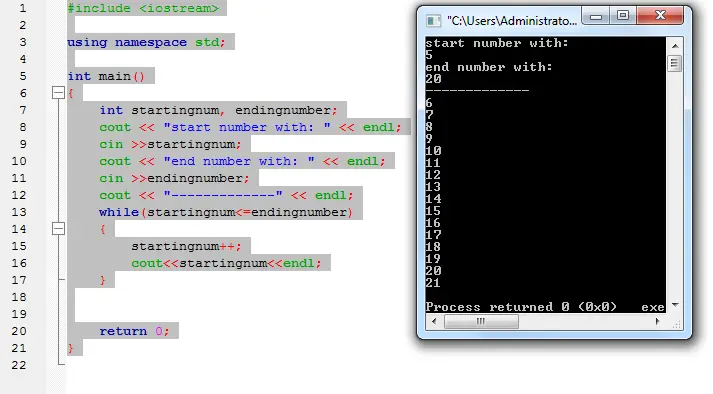While Loop in C++

Another loop in c++ or in any programming languages is the while loop. This kind of loop will just repeat the line of codes in a number given in the expression.

The discussion and the step by step tutorial will be posted on inettutor.com.

## User Defined Function in C++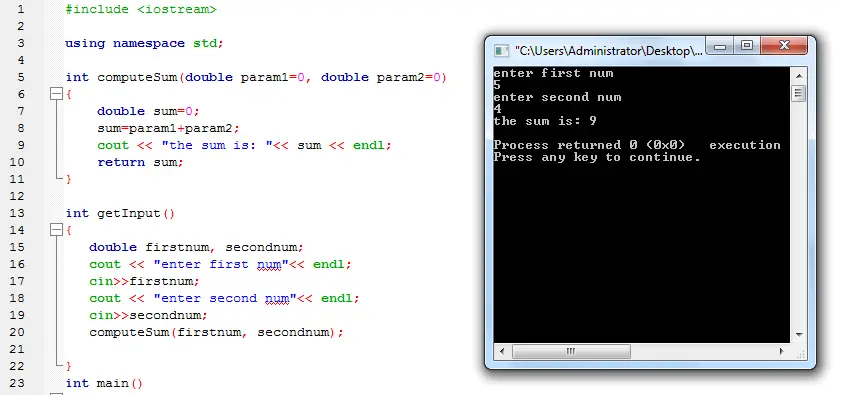User Defined Function in C++

User defined simply means created by the programmer to execute a certain command. Programming languages has its own built-in functions that are ready to use but programmers can also create functions that will fit their needs. This is actually the most challenging aspect of programming, how to understand and create your own functions and this program might help you (for beginners).

Happy Coding!

## Switch Statement in C++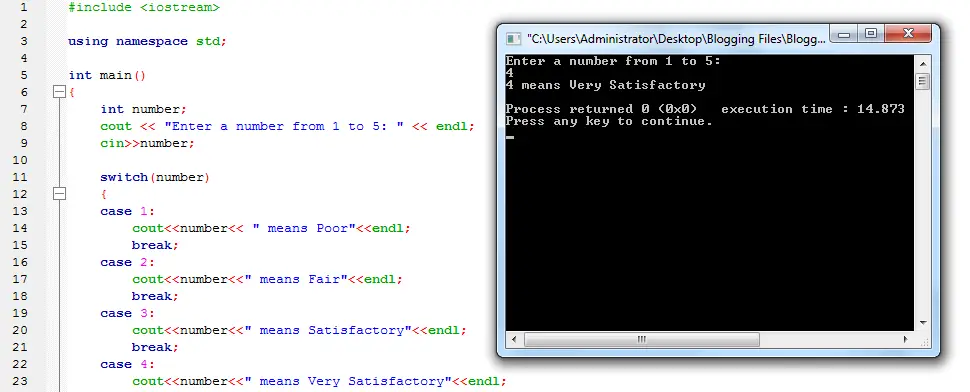Switch Statement in C++

A program in c++ that showcase the use and function of a switch statement. Switch statement is just like if elseif statement, what it does is to execute a statement that matches the expression’s value. If it has no match then the default case will be executed.

## Sum of Numbers in an Array in C++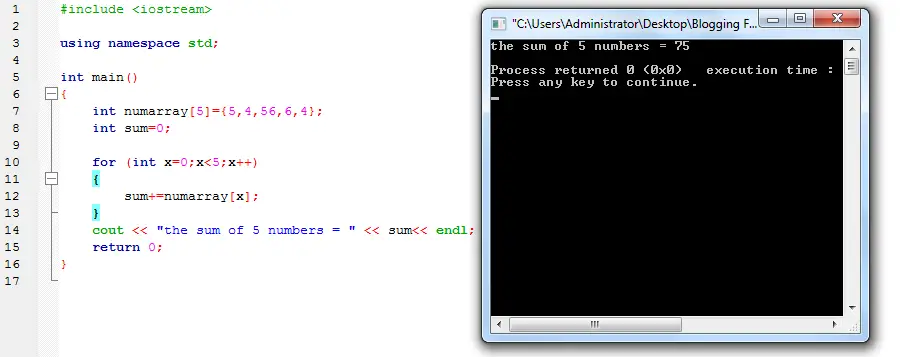Sum of Numbers in an Array in C++

A sample program in c++ that will compute the sum of numbers in an array. The program will compute and display the sum of 5 numbers in an array. The program uses for loop to compute the sum and stops the loop when the condition is met.

## Combine Strings in C++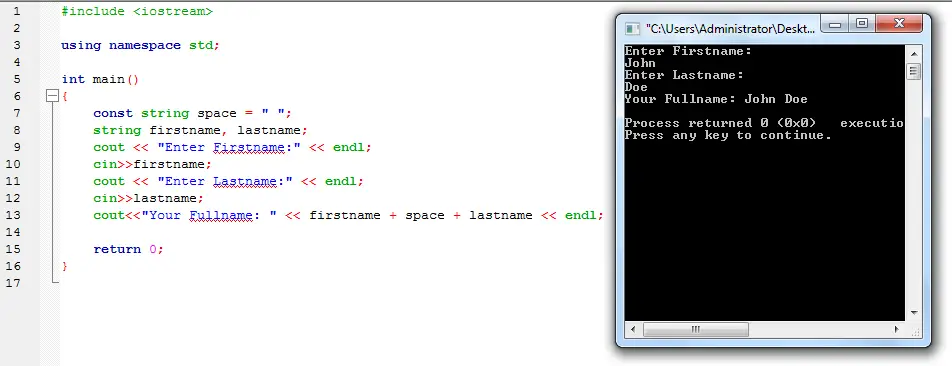Combine Strings in C++

Concatenation is a proper term for combining two or more strings. This c++ program will ask the user to enter a firstname and lastname, the output will be the combination the firstname and lastname. The operator used to combine two strings is the plus sign “+”.

## C++ sizeof Operator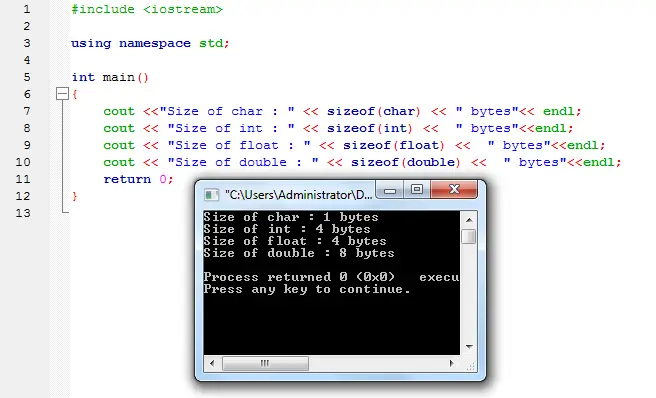C++ sizeof Operator
sizeof Operator will return a number of bytes in a variable or data type. This c++ program will display the size of 4 datatypes (char, int, float and double) in bytes.

Open the main.cpp in codeblocks or whatever ide you are using that supports c++.

## Number of Respondents Calculator in C++Number of Respondents Calculator in C++

Sample program in c++ that will compute the number of respondents using the sloven’s formula. To compute the number of respondents the user must enter the total population size.

Sloven’s formula:
n = N/(1+(Ne2))
where:
n = number of respondents
N = total population
e = margin of error , 0.05 in this case

## Random Numbers in C++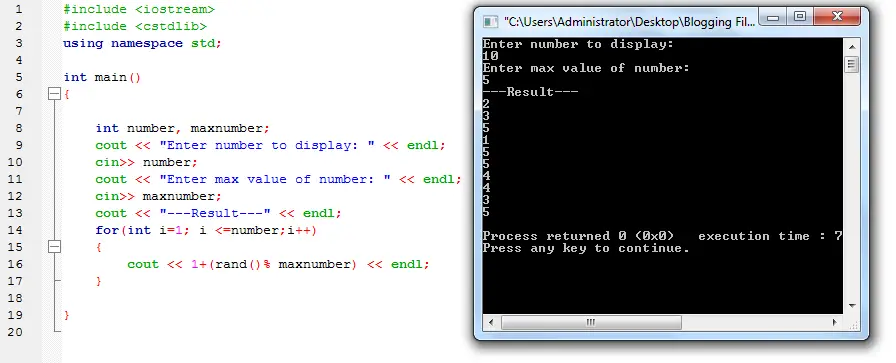Random Numbers in C++

A program in c++ that generates random numbers using rand function. The user will need to input how many numbers he/she want to display and the max value of the number.

The numbers entered will be then executed inside a for loop and random numbers will displayed.
Happy Coding!

## Get length of a string in C++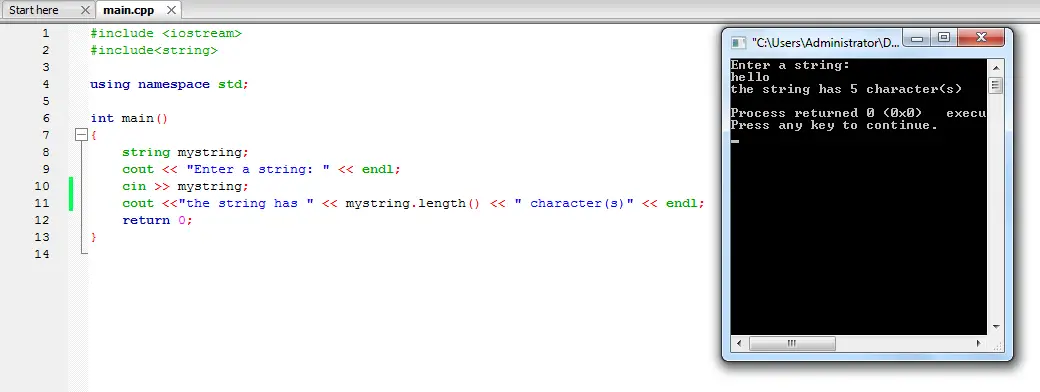Get length of a string in C++

A c++ program that will count the number of characters in a given string. The program will first ask the user to enter a string, then the length() property will count the number of characters in a string object.

Open the main.cpp in codeblocks or any ide that supports c++.